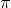## 2. A direular para is to be divided into 12 equal sectors. What is the radian measure of the angles. based from the terminal side

Question

2. A direular para is to be divided into 12 equal sectors. What is the radian measure of the angles.
based
from the terminal side for every slice of the pizza? Draw an exact diagram and label each
terminal side with the equivalent radian
measure .​

in progress 0
2 months 2021-09-02T15:01:50+00:00 1 Answers 2 views 0

1. Step-by-step explanation:

Step 1 Try to draw a perfect circle

Step 2 Divide into 12 equal sections if you can

A circle has 2 pi radians or 360 degrees in it

Divide 2 pi by 12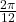Which equal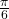For every nth sector you on multiply the nth sector your on by pi/6

For example let say ur on second sector.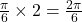orLet say ur on the 6th sector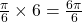or